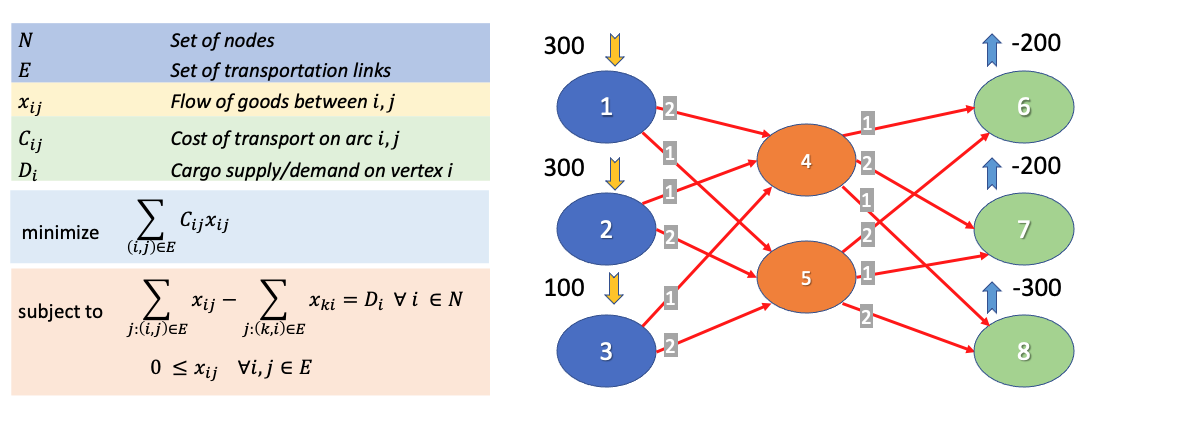# Notebook 3.4 - Minimum Cost Flow (Transhipment) using PuLP¶

Let us now attempt to model the Transhipment or Minimum Cost Flow Problem, that we also covered in our session videos.### Setting up¶

In [ ]:
from pulp import *

prob = LpProblem('prob', LpMinimize)


### Entry of problem instance data¶

On our previous notebook, we created our objects one by one, through the declation of two arrays that contained all the objects that we needed to create.

S = ['S1', 'S2', 'S3']
D = ['D1', 'D2', 'D3']

For this particular model however, we are going to try something else:

In [ ]:
N = [str(i+1) for i in range(8)]
N


The above declaration created 8 node elements in our array, which were generated using an in-line for loop. For every value in a range of integers between 0 and 8, the str(i+1) command was adding a stringified version of the value, incremented by one.

The increment is essential if we want the list of node names to start from 1, since the range(8) command would return a series of 8 values, starting from 0. You can check this on your own below:

In [ ]:
list(range(8))


Our list of supply/demand values is provided in a more "traditional" way. We use positive values to indicate that a node is a node supplier, null values to indicate transhipment nodes, and negative values to indicate demand nodes.

The values will be provided in the same sequence as the node IDs.

In [ ]:
supply = [300, 300, 100, 0, 0, -200, -200, -300]


We can now convert this into a dictionary of values, with node IDs used as keys.

In [ ]:
D = dict(zip(N, supply))
D


The nested dictionary of link costs is defined in a similar way as the one we discussed in our previous notebook.

In :
C = {'1': {'4': 2, '5': 1},
'2': {'4': 1, '5': 2},
'3': {'4': 1, '5': 2},
'4': {'6': 1, '7': 2, '8': 1},
'5': {'6': 2, '7': 1, '8': 2}}


Our set of links is also created using using inline loop.

In :
E = [(i,j) for i in N for j in N if i in C.keys() if j in C[i].keys()]
E

Out:
[('1', '4'),
('1', '5'),
('2', '4'),
('2', '5'),
('3', '4'),
('3', '5'),
('4', '6'),
('4', '7'),
('4', '8'),
('5', '6'),
('5', '7'),
('5', '8')]

I appreciate that inline loops are a bit confusing at first. Let's try to recreate this result using a traditional loop.

In :
E1 = []

for i in N:                        # for every node i in set N
for j in N:                    # for every node j in set N
if i in C.keys():          # if we have an inner dictionary in C for the costs of links beginning in i
if j in C[i].keys():   # and if that inner dictionary contains an entry for the node pair i,j
E1.append((i,j))   # then a link between i and j exists, and we will an entry to our set of links.

E1

Out:
[('1', '4'),
('1', '5'),
('2', '4'),
('2', '5'),
('3', '4'),
('3', '5'),
('4', '6'),
('4', '7'),
('4', '8'),
('5', '6'),
('5', '7'),
('5', '8')]

And finally, we can declare our decision variables

In :
x = LpVariable.dicts('x', E, lowBound = 0)


### Objective and constraints¶

We will ne using the same objective function as before:

In :
prob += lpSum([C[i][j] * x[i,j] for (i,j) in E])


Even though our flow conservation constraint might look a little bit intimidating on paper, I believe that it looks a lot more straightforward when transliterated into a PuLP command!

In :
for i in N:
prob += (lpSum([x[i,j] for j in N if (i,j) in E]) - \
lpSum([x[k,i] for k in N if (k,i) in E])) == D[i]


### Obtaining a solution¶

In :
status = prob.solve()

print('SOLUTION:')
for v in prob.variables():
print(f'\t\t{v.name} = {v.varValue}')

print('\n') # Prints a blank line
print(f'OBJECTIVE VALUE: {prob.objective.value()}')

SOLUTION:
x_('1',_'4') = 0.0
x_('1',_'5') = 300.0
x_('2',_'4') = 300.0
x_('2',_'5') = 0.0
x_('3',_'4') = 100.0
x_('3',_'5') = 0.0
x_('4',_'6') = 100.0
x_('4',_'7') = 0.0
x_('4',_'8') = 300.0
x_('5',_'6') = 100.0
x_('5',_'7') = 200.0
x_('5',_'8') = 0.0

OBJECTIVE VALUE: 1500.0


## Summary¶

from pulp import *

prob = LpProblem('prob', LpMinimize)

# INSTANCE DEFINITION

supply = [300, 300, 100, 0, 0, -200, -200, -300]

N = [str(i+1) for i in range(8)]

D = dict(zip(N, supply))

C = {'1': {'4': 2, '5': 1},
'2': {'4': 1, '5': 2},
'3': {'4': 1, '5': 2},
'4': {'6': 1, '7': 2, '8': 1},
'5': {'6': 2, '7': 1, '8': 2}}

E = [(i,j) for i in N for j in N if i in C.keys() if j in C[i].keys()]

# DECISION VARIABLE GENERATION

x = LpVariable.dicts('x', E, lowBound = 0)

# PROBLEM FORMULATION
prob += lpSum([C[i][j] * x[i,j] for (i,j) in E])

for i in N:
prob += (lpSum([x[i,j] for j in N if (i,j) in E]) - \
lpSum([x[k,i] for k in N if (k,i) in E])) == D[i]

# SOLUTION
status = prob.solve()

print(f'STATUS\n{LpStatus[status]}\n')

print('SOLUTION:')
for v in prob.variables():
print(f'\t\t{v.name} = {v.varValue}')

print('\n') # Prints a blank line
print(f'OBJECTIVE VALUE: {prob.objective.value()}')